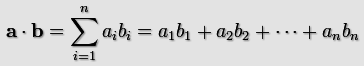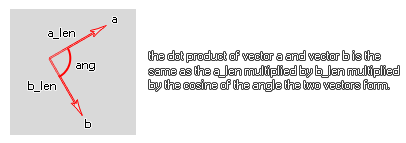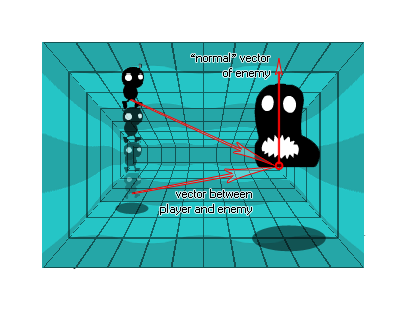## dot_product_3d

### 语法：

dot_product_3d(x1, y1, z1, x2, y2, z2)

x1 第一个向量的x坐标。
y1 第一个向量的y坐标。
z1 第一个向量的z坐标。
x2 第二个向量的x坐标。
y2 第二个向量的y坐标。
z2 第二个向量的z坐标。

### 描述a · b = (x1*x2) + (y1*y2) + (z1*z2);

a · b = (length of a) * (length of b) * cos(angle)• 如果 a 和 b 是垂直的 (在90度之间), 点积的结果将是零, 因为 cos (ang) 为零。
• 如果 a 和 b 之间的角度小于 90度, 点乘积将为正 (大于零), 因为 cos (ang) 将为正。
• 如果 a 和 b 之间的角度大于 90度, 点乘积将为负 (小于零), 因为 cos (ang) 将为负。Basically, we are getting the vector normal from the enemy perpendicular to the floor and then we are getting the vector of the player to the enemy. We will then get the dot_product of these vectors, and if the result is positive the player is "above" the enemy floor plane and if it is negative he is below. 下面的示例中提供了要完成此工作的实际代码。

### 例如：

var x1, y1, x2, y2;
x1 = 0;
y1 = 1;
z1 = 0;
x2 = o_Player.x - x;
y2 = o_Player.y - y;
z2 = o_Player.z - z; if dot_product_3d(x1, y1, z1, x2, y2, z2) > 0 above=true else above=false;